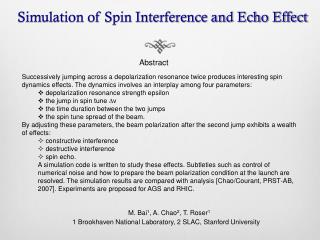DownloadDownload PresentationSimulation of Spin Interference and Echo Effect

# Simulation of Spin Interference and Echo Effect

Télécharger la présentation## Simulation of Spin Interference and Echo Effect

- - - - - - - - - - - - - - - - - - - - - - - - - - - E N D - - - - - - - - - - - - - - - - - - - - - - - - - - -
##### Presentation Transcript

1. Simulation of Spin Interference and Echo Effect • Abstract • Successively jumping across a depolarization resonance twice produces interesting spin dynamics effects. The dynamics involves an interplay among four parameters: • depolarization resonance strength epsilon • the jump in spin tune ∆ν • the time duration between the two jumps • the spin tune spread of the beam. • By adjusting these parameters, the beam polarization after the second jump exhibits a wealth of effects: • constructive interference • destructive interference • spin echo. • A simulation code is written to study these effects. Subtleties such as control of numerical noise and how to prepare the beam polarization condition at the launch are resolved. The simulation results are compared with analysis [Chao/Courant, PRST-AB, 2007]. Experiments are proposed for AGS and RHIC. • M. Bai1, A. Chao2, T. Roser1 • 1 Brookhaven National Laboratory, 2 SLAC, Stanford University

2. Introduction • While the spin component in the horizontal plane precesses around the vertical direction, the vertical component is given by Spin motion at the vicinity of a depolarizing resonance ε

3. Interference-overlap effect of multi resonance crossing • For single pt, the vertical component of final polarization is Polarization after crossing a resonance twice depends on the spin phase advance Ω(θ-θ1) between the two crossings.

4. Interference-overlap effect of multi resonance crossing • multiple particles with Gaussian • distributed momentum spread • σE/E=0.0002 • ε0=0.005, A=0.01, jump size:0.02 θ1 θ2 • Constructive case Destructive case

5. Spin Echo Effect • For a beam of polarized particles with a momentum spread of σδ in Gaussian distribution A sudden spin signal occurs at θ2 −θ1 after the second resonance jump Shock response from the 1st resonance jump. It precess with frequency Ω

6. Spin Echo Effect A sudden spin signal occurs at θ2 −θ1 after the second resonance jump • Condition: • interference terms are insigficant: • Jump length: • The magnitude of echo signal Spin jump is within resonance width

7. Numerical Simulation of Spin Echo Effect • beam energy Gγ=4.6 • Single resonance is induced by an rotating field with a constant spin kick around an axis which rotates at a fixed frequency • a total of 1201 particles with an rms energy spread • resonance is adiabatically turned on to a strength 0.002 at a fixed tune of 0.599. The jump size is 0.002

8. Simulation result 40,000 turns between two jumps

9. Smearing of Spin Echo Effect • The echo signal is significant damped if • The echo signal can be smeared out due to high order dependence of omega on the momentum spread, i.e.

10. Simulation of Spin Echo Smearing 300,000 turns

11. Observe echo at AGS • Beam energy: Gγ=4.6 • AGS An ac dipole at frequency ~100kHz and 20 Gauss-m • ε0 = 0.0001 • This requires: • A=0.00005 • Momentum spread: σδ~ 4x10-6 • This is very difficult to obtain • In addition, the oscillating field of single ac dipole drives two resonances instead of one. This introduces additional motions

12. Oscillating field vs. Rotating field • Two ac dipoles with a 90 degrees spin rotator in the middle to provide a rotating field. Each ac dipole was adiabatically ramped to 333 Gauss-m • A single ac dipole was adiabatically ramped to 333Gauss-m with a fixed tune at 0.01 away from spin tune at Ggamma=4.6

13. Possible experiments at RHIC • RHIC spin flipper provides a rotating field with a maximum strength of ε0 = 0.00092 • Small spin tune spread because the two full snakes which removes the spin tune dependence on the beam energy • Source of Spin tune spread • difference of horizontal divergence of the beam at the two snakes. This causes a spread of horizontal angle between the two snakes. The spin tune spread is given by Beam emittance Twiss parameter at snakes

14. Simulation of Spin Echo in the presence of full snakes • two snakes apart by 180 degrees • spin tune spread is achieved through the spread of • the angle between the two snake axis • 1201 particles. ε0= 0.00092, A=0.00046 • spin tune spread 4d-5 • Tune jump from 0.49956 to 0.50048 in 200

15. Conclusion • The interference-overlap effect when a single resonance is jump crossed twice was studied through numerical simulation. The single resonance excited by a rotating field which consists of two ac dipoles and a 90 degree spin rotator in between. • Simulation of using the same rotating field in the presence of two full Siberian snakes also shows an spin echo effect can be generate if the condition is met. This suggests that it may be possible to observe the spin echo effect at RHIC injection • Simulation of using single ac dipole was also done and shows that the extra term excited by the oscillating field introduces extra noises to the spin signal • Work to be done in the future: • Detailed simulation using RHIC lattice • Design and propose experiment at RHIC